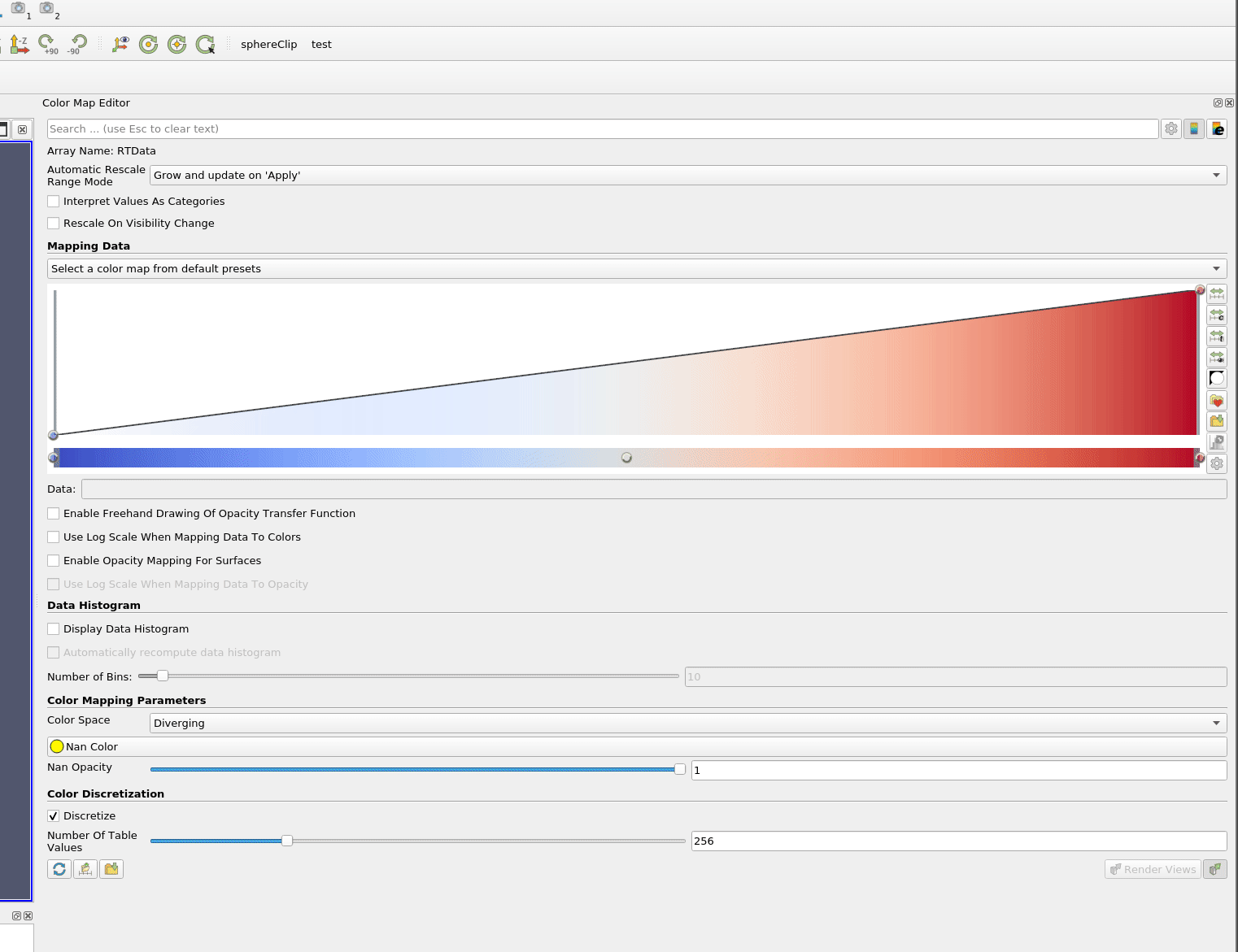# Color Map to png file

I am looking for a way via python (simple.py) to extract an image of the color map. Any pointers on how I might do this for say cool2warm? I just need access to the raw values of the table.

do you want an image or the values ?

Ultimately, I want to be able to create images of arbitrary sizes of any color map in paraview. I assume that the 'color discretization / number of values` allows me to control the underlying size of the lookup table, so if I can get access to that array of rgb values, then I can make images of any size. That said, I haven’t figured out how to get access to the array yet.

Yes

Well you can do that by just controlling the style of the color map, here is a state file to demonstrate it:

cm.pvsm (313.7 KB)

but only the color table values.

I do not understand, do you want an image with colors or the values of the color map in a text form ?

I want an array of rgb values.

Here is how to do it:Thanks for responding.

Let me try thing again. I am assuming the cool2warm color table is discretized into say 256 values (since there is an option in the gui that asks me how many bins I want to discretize the cmap into), where the 0 index maps to the beginning of the normalized range (i.e.,m [0,1]). 255 maps to 1. Those are the values I am interested in getting, not the RGB points that define the color map in the diverging color space.

I’m afraid these discretized values can’t be recovered from the interface of ParaView.

Even in python?

Yes, the actual discretized values are only computed at the rendering step.

That being said, this discretization is trivial, you can just compute it from the initial RGB values.

So just implement the six supported color spaces in python?

For anyone wondering:

``````    lut = display.LookupTable.GetClientSideObject()

dataRange = customRange
if not dataRange:
dataRange = lut.GetRange()

delta = (dataRange - dataRange) / float(numSamples)

colorArray = vtkUnsignedCharArray()
colorArray.SetNumberOfComponents(3)
colorArray.SetNumberOfTuples(numSamples)

rgb = [ 0, 0, 0 ]
for i in range(numSamples):
lut.GetColor(dataRange + float(i) * delta, rgb)
r = int(round(rgb * 255))
g = int(round(rgb * 255))
b = int(round(rgb * 255))
colorArray.SetTuple3(i, r, g, b)``````
2 Likes